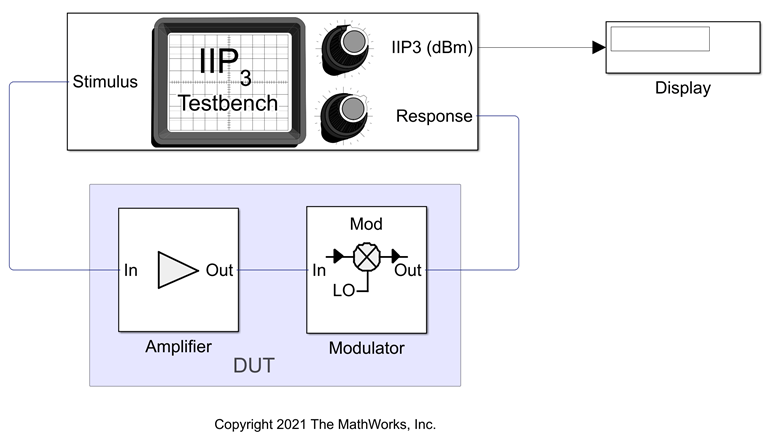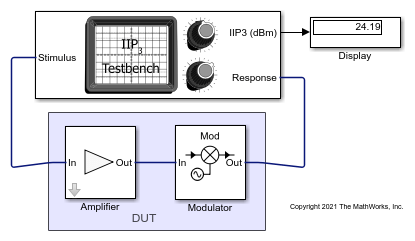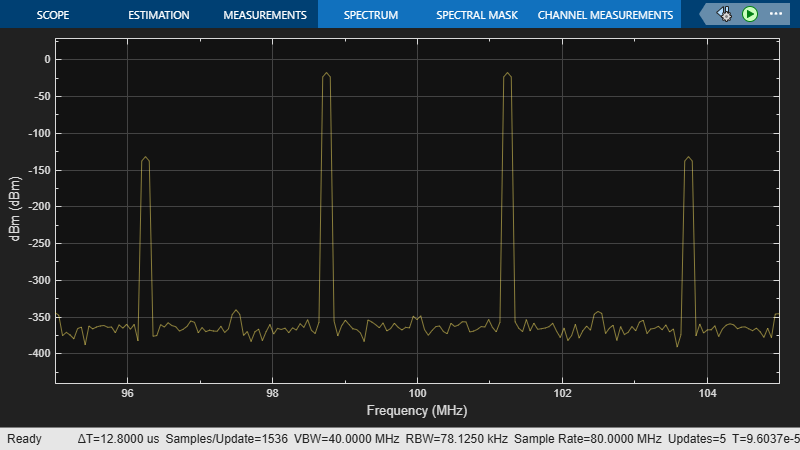Measure IIP3 of Device Under Test

Use the IIP3 Testbench block to measure the input third-order intercept (IIP3) of device under test (DUT).

Connect the blocks as shown in the model.Set the parameters for DUT and the testbench.

Amplifier block:

• Available power gain10 dB

• Intercept points conventionInput

• IP332 dBm

Mixer block:

• Available power gain5 dB

• Local oscillator frequency2.0 GHz

• Intercept points conventionInput

• IP335 dBm

• Filter typeHighpass

• ImplementationConstant per carrier

• Passband edge frequency2.0 GHz

IIP3 Testbench block:

• Input frequency (Hz)2.1e9

• Output frequency (Hz)0.1e9

• Simulate noise (both stimulus and DUT internal)off

Run the model. You will see that the display shows an IIP3 value of 24.19 dBm. This value can be verified analytically using the equation provided in .

IIP3 of the DUT = – 10*log10(1/10^((32)/10) + 10^(10/10)/10^((35)/10)) = 24.2099 dBm

where,

IIP3 of the amplifier in linear scale = 10^((32)/10)

IIP3 of the mixer in linear scale = 10^((35)/10)

Available power gain of the amplifier = 10^(10/10)Razavi, Behzad. “Basic Concepts “ in RF Microelectronics, 2nd edition, Prentice Hall, 2012.